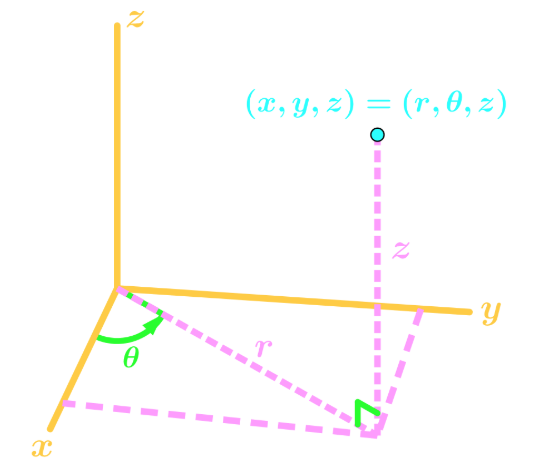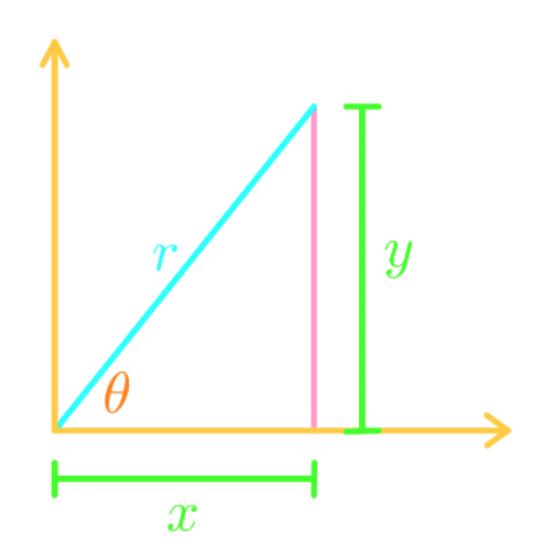# Cylindrical coordinates

### Cylindrical coordinates

#### Lessons

Notes:

Instead of Cartesian coordinates, we use cylindrical coordinates for situations you will see in future sections.
For now, we will just learn how to convert from 3D Cartesians coordinates to 3D cylindrical coordinates, and vice versa. In other words,

$(x,y,z) \to (r, \theta, z )$

Suppose we have the following graph:Since the z coordinate doesn't change from converting, we will just focus on the x and y. Thus, we make it into 2D graph:From this graph, we can get the following equations:

$x = r\cos\theta$
$y = r\sin\theta$
$x^2 + y^2 = r^2$
$\tan^{-1} (\frac{y}{x}) = \theta$

These equations will be useful when converting 3D coordinates.
• Introduction
Cylindrical Coordinates Overview:
a)
What are Cylindrical Coordinates?
• Instead of (${x}, {y}, {z}$), we have (${r},\theta, {z}$)
• Graph of the coordinates in 3D

b)
Equations to Convert from Cartesian to Cylindrical
• Finding the Equations
• Ignoring ${z}$
• Trig Ratios, Angles, and Pythagoras Theorem

c)
Example of Converting Equations
• Cartesian to Cylindrical
• Cylindrical to Cartesian

• 1.
Convert the following Cartesian point into Cylindrical Coordinates:

$(1,3,5)$

• 2.
Convert the following Cartesian point into Cylindrical Coordinates:

$(-2,1,3)$

• 3.
Converting Cartesian Equations into Cylindrical Coordinates
Convert the following Cartesian Equation into Cylindrical Coordinates:

$x+y^2 + z^3 = -(x^2)$

• 4.
Convert the following Cartesian Equation into Cylindrical Coordinates:

$\frac{x^2y}{z} = yz$

• 5.
Converting Cylindrical Equations into Cartesian Coordinates
Convert the following Cylindrical Equation into Cartesian Coordinates:

$r^2 + 4\theta = z$

• 6.
Convert the following Cylindrical Equation into Cartesian Coordinates:

$\sin\theta + \cos\theta = 2z$

• 7.
Graphing the Cylindrical Equations by Changing into Cartesian Coordinates
Graph the Cylindrical Equation in the Cartesian Plane:

$r^2 - 4r\cos\theta = 14$